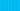PricingResources

# What is depreciation in accounting?

Written by

While small businesses can write off expenses as and when they occur, it’s not possible to expense larger items – also known as fixed assets – such as vehicles or buildings. That’s where depreciation, an accounting method you can use to spread the value of an asset over multiple years, comes in. But what does depreciation mean? Find out everything you need to know about the different types of depreciation, right here.

## What does depreciation mean?

Depreciation is what happens when assets lose value over time until the value of the asset becomes zero, or negligible. Depreciation can happen to virtually any fixed asset, including office equipment, computers, machinery, buildings, and so on. One fixed asset that is exempt from depreciation is the value of land, which appreciates (increases) over time.

## How to calculate depreciation

There are three main depreciation methods that anyone trying to find out how to calculate depreciation should familiarise themselves with. We’ll explore these different types of depreciation in the next section. But before you can start to work out depreciation, you’ll need to know a couple of key pieces of information:

• Useful life – This is essentially the length of time that an asset is considered to be productive. Beyond its useful life, it’s no longer cost-effective to continue using the asset.

• Salvage value – After the useful life of the asset has concluded, you may wish to sell the asset at a reduced rate. This is referred to as the salvage value of the asset.

• Cost of asset – This is the full cost of the asset, including taxes, setup expenses, and shipping.

By including depreciation in your accounting records, your business can ensure that it records the right profit on the balance sheet and income statement. As depreciation is a highly complex area, it’s always a good idea to leave it to the experts. Ensure that your company’s accountant handles all calculations relating to depreciation. In addition, accounting software like Xero can do the maths automatically.

## Types of depreciation

There are a couple of different depreciation methods that you can use. Here’s a quick rundown of the three main types of depreciation:

### 1. Straight-line depreciation

This is the simplest depreciation method. Essentially, the value of the asset depreciates by the same amount each year, until it reaches zero. So, if an asset has a useful life of 10 years, its value would depreciate by 10% every year. You can calculate straight-line depreciation using the following formula:

### 2. Units of production depreciation

In some cases, it makes more sense to calculate depreciation by measuring the work the asset does, rather than the time it serves. So, in this depreciation method, equal expense rates are assigned to each unit of production, meaning that depreciation is based on output capacity rather than number of years. There are two steps you’ll need to go through to calculate units of production depreciation.

Firstly, you need to calculate the per-unit depreciation:

Then, you’ll need to calculate the total depreciation, based on the actual units that have been produced:

### 3. Double declining depreciation

Double declining depreciation is an accelerated form of depreciation, where a higher percentage of value is lost in the early stages of the asset’s useful life. This is particularly useful when assets are consumed more rapidly during the first few years. You can calculate double declining depreciation as follows:

## Why does depreciation matter?

Ultimately, depreciation accounting gives you a much better understanding of the true cost of doing business. To gain a more accurate picture of your company’s profitability, you’ll need to know depreciation, because as assets wear down and become less valuable, they’ll need to be replaced. Depreciation helps you understand how much value your assets have lost over the years, and if you don’t factor it into your revenue, it could mean that you’re underestimating your costs.

In addition, depreciation plays a key role in tax. Put simply, lower profits = lower taxes. If you aren’t accounting for depreciation, you could end up paying more tax. Gradually, you may be able to claim the entire value of a particular asset off your taxes. Depreciation is also important for valuing your business, as a depreciation in the value of your assets could mean that your business loses value as well. Plus, assets are often used to secure financing, so as they lose value, you may find it harder to get a loan.

## We can help

GoCardless helps you automate payment collection, cutting down on the amount of admin your team needs to deal with when chasing invoices. Find out how GoCardless can help you with ad hoc payments or recurring payments.

Sales

Support

+61 3 8375 9198